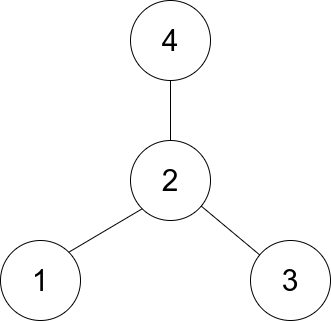# [Leetcode]1791. Find Center of Star Graph

limelimejiwon·2022년 5월 18일
0

## Python으로 공부하는 코딩테스트

목록 보기
54/67## 📄 Description

There is an undirected star graph consisting of n nodes labeled from 1 to n. A star graph is a graph where there is one center node and exactly n - 1 edges that connect the center node with every other node.

You are given a 2D integer array edges where each $edges[i] = [{u_i}, {v_i}]$ indicates that there is an edge between the nodes ${u_i}$ and ${v_i}$. Return the center of the given star graph.

### Example 1:Input: edges = [[1,2],[2,3],[4,2]]
Output: 2
Explanation: As shown in the figure above, node 2 is connected to every other node, so 2 is the center.

### Example 2:

Input: edges = [[1,2],[5,1],[1,3],[1,4]]
Output: 1

### Constraints:

• $3 <= n <= {10^5}$
• edges.length == n - 1
• edges[i].length == 2
• 1 <= ${u_i}$, ${v_i}$ <= n
• ${u_i}$ != ${v_i}$
• The given edges represent a valid star graph.

## 💻 My Submission

class Solution:
def findCenter(self, edges: List[List[int]]) -> int:
_dict=defaultdict(list)
for n1,n2 in edges:
_dict[n1].append(n2)
_dict[n2].append(n1)
for k,v in _dict.items():
if len(_dict[k])==len(edges):
return k

References